Practice the AP 10th Class Physical Science Bits with Answers Chapter 4 Refraction of Light at Curved Surfaces on a regular basis so that you can attempt exams with utmost confidence.

## AP State Syllabus 10th Class Physical Science Bits 4th Lesson Refraction of Light at Curved Surfaces with Answers

CONCEPT – I : Refraction of Light at A Single Curved Surface

Question 1.
What happens to a ray that travels along the principal axis, strikes a convex surface and passes from rarer to denser medium ?
A) The ray deviates from its path
B) The ray does not deviate from its path
C) The ray bends towards the normal after refraction
D) The ray bends away from the normal after refraction
B) The ray does not deviate from its path

Question 2.
What happens to a ray that travels along the principal axis, strikes a concave surface and passes from rarer to denser medium?
A) The ray deviates from its path
B) The ray does not deviate from its path
C) The ray bends towards the normal after refraction
D) The ray bends away from the normal after refraction
B) The ray does not deviate from its pathQuestion 3.
What happens to a ray that travels through the centre of curvature of a single curved surface ?
A) The ray deviates from its path
B) The ray does not deviate from its path
C) The ray bends towafds the normal
D) The ray bends away from the normal
B) The ray does not deviate from its path

Question 4.
The refracted ray …………….. when a ray travelling parallel to the principal axis, strikes a convex surface and passes from rarer to denser medium
A) moves towards the principal axis
B) moves away from the principal axis
C) moves parallel to the principal axis
D) moves along the normal
A) moves towards the principal axis

Question 5.
The refracted ray ………………. when a ray travelling parallel to the principal axis, strikes a convex surface and passes from denser medium to rarer medium
A) moves towards the principal axis
B) moves away from the principal axis
C) moves parallel to the principal axis
D) moves along the normal
B) moves away from the principal axis

Question 6.
The refracted ray ………………. when a ray travelling parallel to the principal axis, strikes a concave surface and passes from rarer medium to denser medium
A) moves towards the principal axis
B) moves away from the principal axis
C) moves parallel to the principal axis
D) moves along the normal
B) moves away from the principal axis

Question 7.
The refracted ray ……………… when a ray travelling parallel to the principal axis, strikes a concave surface and passes from denser medium to rarer medium
A) moves towards the principal axis
B) moves away from the principal axis
C) moves parallel to the principal axis
D) moves along the normal
A) moves towards the principal axis

Question 8.
Trace out the correct diagram from the following.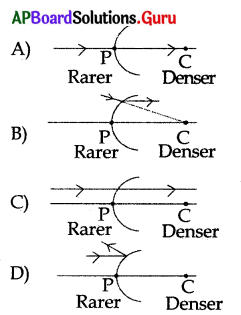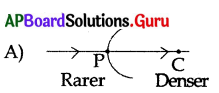Question 9.
Trace out the correct diagram from the following.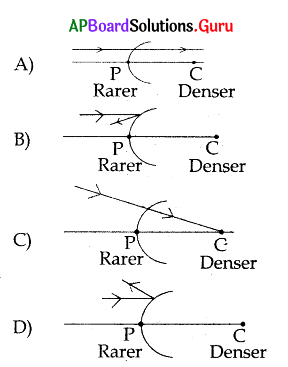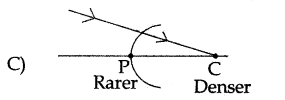Question 10.
Trace out the correct diagram from the following.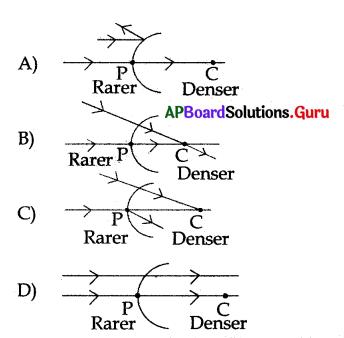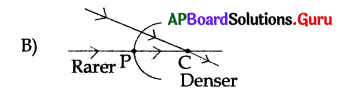Question 11.
Trace out the correct diagram from the following. When a ray of light travels parallel to the principal axis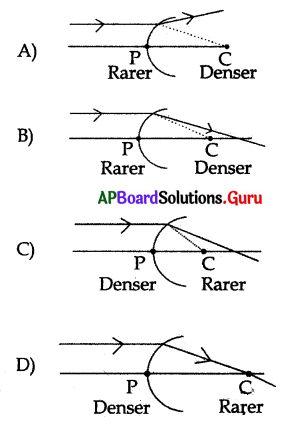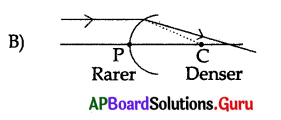Question 12.
Trace out the correct diagram from the following when a ray of light travels parallel to the principal, axis.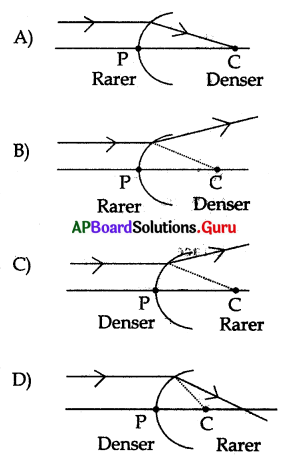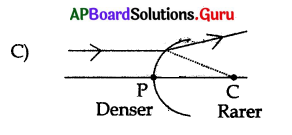Question 13.
Trace out the correct diagram from the following when a ray of light travels parallel to the principal axis.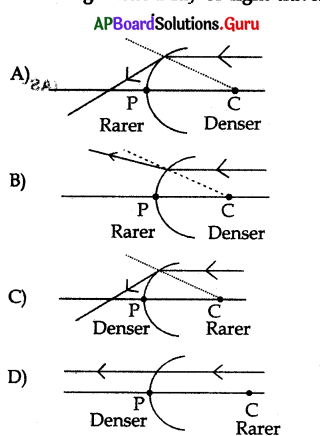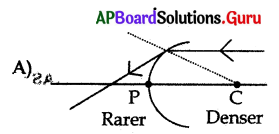Question 14.
The formula used when a light ray enters a medium with refractive index n2 from a medium with refractive index n1 at curved surface with a radius of Curvature R is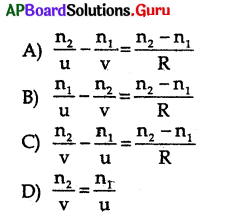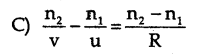Question 15.
The formula used when a light ray enters a medium with refractive index n2 from a medium with refractive index n1 at plane surface is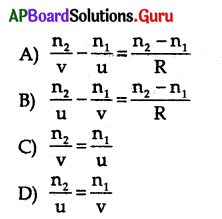C) $$\frac{n_{2}}{v}=\frac{n_{1}}{u}$$

Question 16.
A bird is flying down vertically to wards the surface of water in a pond with constant speed. There is a fish inside the water. If the fish is exactly vertically below the bird, then the bird will appear
to the fish to be
a) farther away than its actual distance
b) closer than its actual distance
c) moving faster than its actual speed
d) moving slower than its actual speed
Which of the above options are true?
A) only (a)
B) (a) and (c)
C) (a) and (d)
D) (b) and (d)
B) (a) and (c)

Question 17.
A person is standing on the bank of a river. A fish inside water will see the person to be
A) taller
B) shorter
C) original height
D) none
A) tallerQuestion 18.
A transparent sphere of radius R and refractive index n is kept in Air.At what distance from the surface of the sphere should a point object be placed on the principal axis so as to form a real image at the same distance from the second surface of the sphere?
A) $$\frac{\mathrm{n}-1}{\mathrm{R}}$$
B) (n – 1).R
C)R+(n – 1)
D)R/n – 1
D)R/n – 1

Question 19.
A lemon kept in a glass of water appears to be bigger than its actual size. This is due to ……….
A) reflection
B) refraction
C) total internal reflection
D) dispersion
B) refraction

Question 20.
A curved surface of radius of curvature R is separating the two media of refractive indices n1 and n2. If u and υ are object and image distances respectively, then the relation is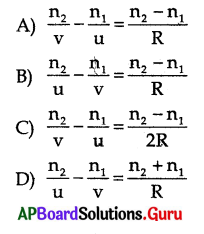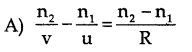CONCEPT – II : Refraction of Light Through “Lenses”

Question 21.
A transparent material is bounded by two surfaces of which one (or) both – surfaces are spherical is called …………………….
A) glass slab
B) mirror
C) prism
D) lens
D) lens

Question 22.
A lens has ……………… curved surfaces.
A) one
B) two
C) A and B
D) zero
C) A and B

Question 23.
A lens which has thick at the middle and thinner at the edges is called ……………………
A) bi convex lens
B) bi concave lens
C) piano – concave lens
D) micro lens
A) bi convex lens

Question 24.
A lens is bounded by two spherical surfaces, thin at the middle and thicker at the edges is called …………………..
A) bi convex lens
B) bi concave lens
C) piano – concave lens
D) piano – convex lens
B) bi concave lensQuestion 25.
Match the following.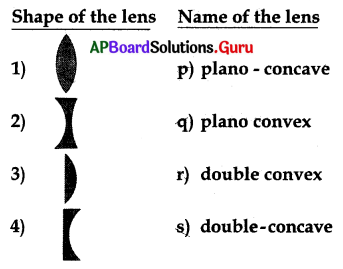A) 1 → p,2 → q,3 → r,4 → s
B) 1 → s,2 → r,3 → q,4 → p
C) 1 → r,2 → s,3 → q,4 → p
D) 1 → r, 2 → s,3 → p,4 → q
C) 1 → r,2 → s,3 → q,4 → p

Question 26.
Identify the concavo – convex lens from the following.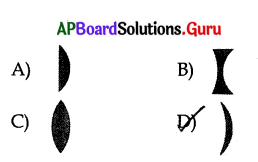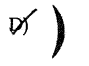Question 27.
The midpoint of a thin lens is called …………………
A) focus
B) centre of curvature
C) optic centre
D) none
C) optic centre

Question 28.
The line joining the centre of curvatures of a lens is called …………………….
A) focal length
C) principal axis
D) prime axis
C) principal axis

Question 29.
The distance between the focal point and optic centre is called …………………
A) focal length
C) principal axis
D) focus
A) focal length

Question 30.
The refracted rays of a parallel beam of light when they are incident on a lens ………………….
A) converges
B) diverges
C) both
D) none
C) both

Question 31.
Which of the following symbol is used to represent convex lens for drawing ray diagrams related to lens ?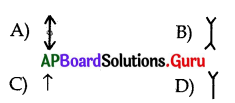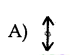Question 32.
Which of the following symbol is used to represent concave lens for drawing ray diagrams related to lens ?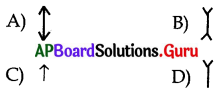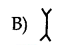Question 33.
The ray passing through the ……………… of the lens is not deviated.
A) optic centre
B) focus
C) centre of curvature
D) none
A) optic centre

Question 34.
Which of the ray does not deviate while passing through the lens ?
A) The ray parallel to the principal axis
B) The ray passing along the principal axis
C) The ray passing through the focus
D) none
B) The ray passing along the principal axis

Question 35.
Statement X : Any ray passing along the principal axis is undeviated.
Statement Y; Any ray passing through the optic centre is undeviated.
A) X and Y are true
B) X and Y ape false
C) X is true, Y is false
D) X is false, Y is true
A) X and Y are true

Question 36.
Which of the following ray diagram is correct?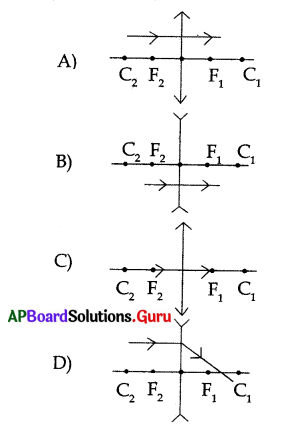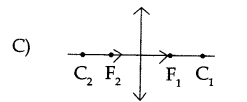Question 37.
Which of the following ray diagram is correct ?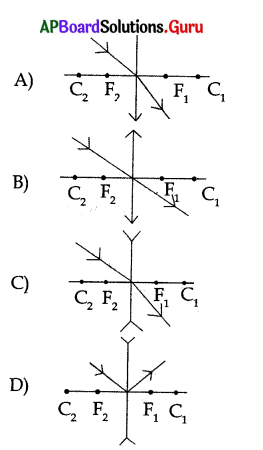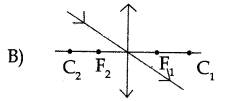Question 38.
The rays which are travelling parallel to the principal axis of a convex lens are ……………….
A) converge at focus
B) converge at centre of curvature
C) appear to diverge from the focus
D) none
A) converge at focus

Question 39.
The refracted rays ………………. when they are pass through the focus of a lens.
A) travel parallel to the principal axis
B) meet at focus
C) meet at centre of curvature
D) none
A) travel parallel to the principal axis

Question 40.
Which of the following ray diagrams is correct ?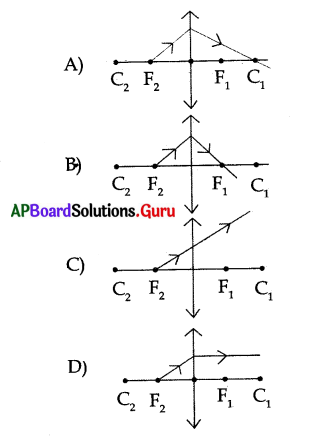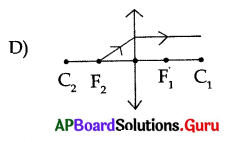Question 41.
What happens when parallel rays of light fall on a lens making some angle with the principal axis ?
A) The rays converge at focus
B) The rays appear to diverge from the focus
C) Both A and B
D) The rays Converge at a point lying on the focal plane
D) The rays Converge at a point lying on the focal plane

Question 42.
The plane perpendicular to the principal axis at the focus is called ………………….
A) Y – axis
B) focal plane
C) nodal plane
D) horizontal plane
B) focal plane

Question 43.
What type of rays fall on the lens when the object is placed at infinity ?
A) parallel rays
B) convergent rays
G) divergent rays
D) none
A) parallel rays

Question 44.
Which one of the following materials cannot be used to make a lens ?
A) water
B) glass
C) plastic
D) clay
D) clay

Question 45.
For a concave lens when object is placed at infinity then the position of the image is …………………..
A) at focus
B) between F and 2F
C) at 2F
D) beyond 2F
A) at focusQuestion 46.
For a convex lens when object is placed beyond C2 then the position of the image is ………………….
A) at F1
B) between F1 and C1
C) at C1
D) beyond C1
B) between F1 and C1

Question 47.
For a convex lens when object is placed at C2 then the position of the image is …………………….
A) at F1
B) between F1 and C1
C) at C1
D) beyond C1
C) at C1

Question 48.
For a convex lens when object is placed between C1 and F2 then the position of the image is …………………
A) at F1
B) between F1 and C1
C) at C1
D) beyond C1
D) beyond C1

Question 49.
For a convex lens when object is placed at F2 then the position of the image is
A) at F1
B) at infinity
C) beyond C1
D) same side of the lens
B) at infinity

Question 50.
For a convex lens when object is placed between focal point and optic centre then the position of the image is …………………
A) at infinity
B) at focus
C) same side of the lens
D) at centre of curvature
C) same side of the lens

Question 51.
For a convex lens where should an object be placed so as to obtain its virtual, erect and magnified image ?
A) at infinity
B) atfocus
C) at centre of curvature
D) between focus and optic centre
D) between focus and optic centre

Question 52.
Where should an object be placed in order to use a convex lens as a magnifying glass ………………….
A) at infinity
B) at focus
C) within the focal length
D) at centre of curvature
C) within the focal lengthQuestion 53.
Where should an object be placed in front of a convex lens so as the image is formed at infinity?
A) at focus
B) between focus and centre of curvature
C) beyond centre of curvature
D) at centre of curvature
A) at focus

Question 54.
Where should an object be placed in front of a convex lens so as to obtain its real, inverted and magnified image?
A) at focus
B) at centre of curvature
C) beyond centre of curvature
D) between focus and centre of curvature
D) between focus and centre of curvature

Question 55.
For what position of an object a real, inverted and same size of image is formed by a convex lens ………………………….
A) at focus
B) at centre of curvature
C) at infinity
D) beyond centre of curvature
B) at centre of curvature

Question 56.
For what position of an object a real, diminished image is formed by a convex lens.
A) at centre of curvature
B) at focus
C) between focus and centre of curvature
D) beyond centre of curvature
D) beyond centre of curvature

Question 57.
For what position of an object a highly diminished size of image is formed at a focus of convex lens.
A) at focus
B) at centre of curvature
C) beyond centre of curvature
D) at infinity
D) at infinity

Question 58.
An object is placed at the following distances from a convex lens of focal length 20 cm. Now match the following.

 Object distance Nature of the image 1) 60 cm a) real and same size of the object 2) 40 cm b) real and magnified image 3) 30 cm c) virtual and magnified image 4) 10 cm d) real and diminished

A) 1 → a, 2 → b, 3 → c, 4 → d
B) 1 → d, 2 → c, 3 → b, 4 → a
C) 1 → b, 2 → a, 3 → b, 4 → c
D) 1 → d, 2 → a, 3 → b, 4 → c
D) 1 → d, 2 → a, 3 → b, 4 → c

Question 59.
If the object is moved towards a convex lens the size of its real image ……………….
A) decreases
B) increases
C) remains the same
D) first decreases and then increases
B) increasesQuestion 60.
A real image formed by a convex lens is always …………………
A) smaller than the object
B) bigger than the object
C) same size as the object
D) none
D) none

Question 61.
A real image formed by a convex lens is always …………………..
A) on the same side of the lens as the object
B) on the other side of the lens as the object
C) erect
D) none
B) on the other side of the lens as the object

Question 62.
An image formed by a convex lens is………………..
A) always virtual
B) always real
C) may be real and virtual
D) always diminished
C) may be real and virtual

Question 63.
A virtual image formed by a convex lens is ………………………
A) erect
B) magnified
C) on the same side of the lens as the object
D) all
D) all

Question 64.
The watch repairer uses a ……………….. lens to see tiny parts of watch.
A) convex lens
B) concave lens
C) both
D) none
A) convex lens

Question 65.
………………. lens are used in making microscopes.
A) Convex lens
B) Concave lens
C) Plane
D) None
A) Convex lens

Question 66.
The minimum possible distance between an object and the real image formed by a convex lens is equal to …………………….
A) zero
B) focal length
D) infinity
A) zero

Question 67.
The maximum possible distance between an object and the real image formed by a convex lens is equal to ………………….
A) zero
B) focal length
D) infinity
D) infinityQuestion 68.
For what position of an obj ect a virtual, erect and diminished image is formed by a concave lens.
A) at focus
B) within the focal length
C) beyond centre of curvature
D) at any position
D) at any position

Question 69.
A concave lens always forms a ………………
A) virtual image
B) erect image
C) diminished image
D) all
D) all

Question 70.
The position of the image formed by a concave lens when the object is placed anywhere between optic centre and infinity is
A) at infinity
B) between optic centre and focus
C) at centre of curvature
D) at focus
B) between optic centre and focus

Question 71.
The position of the image formed by a concave lens when the object is placed at infinity is …………………
A) at focus
B) at infinity
C) at centre of curvature
D) between optic centre and focus
A) at focus

Question 72.
The nature of the image formed by a concave lens when the object is placed in front of it.
A) virtual
B) erect
C) diminished
D) all
D) all

Question 73.
The minimum possible distance between an object and the image formed by a concave lens is ……………………….
A) equal to focal length
B) less than the focal length
C) infinity
D) zero
B) less than the focal length

Question 74.
The maximum possible distance between an object and theimage formed by a concave lens is …………………….
A) equal to focal length
B) less than the focal length
C) zero
D) infinity
D) infinityQuestion 75.
…………….. are used in wide angle spyhole in doors.
A) Concave lens
B) Convex lens
C) Concave mirror
D) Convex mirror
A) Concave lens

Question 76.
The size of the image formed by a convex lens is same as that of the object when the object is placed ……………………..
A) at the centre of the curvature
B) between the centre of curvature and focus
C) beyond the centre of curvature
D) between focus and centre of curvature
A) at the centre of the curvature

Question 77.
The lens which is thin at the middle on both sides and thicker at the edges is …………………….
A) bi – convex
B) concave – convex
C) plano-concave
D) bi concave
D) bi concave

Question 78.
Convex lens is used in the making of microscopes because ………………………
A) it gives diminished image
B) it gives magnified virtual image same side of the object
C) it gives real image behind the screen
D) it gives magnified image opposite side of the lens
B) it gives magnified virtual image same side of the object

Question 79.
The lens which can form real and virtual image in air is …………………..
A) bi convex
B) bi concave
C) plano concave
D) plano convex
A) bi convex

Question 80.
Every lens has …………….. focal points.
A) one
B) two
C) three
D) infinity
B) two

Question 81.
In which one amongthe following cases the convex lens does not give a real image?
A) When the object is placed between the focal point and optic centre
B) When the object is placed beyond the centre of curvature
C) When the object is placed between the centre of curvature and focal point
D) When the object is placed on the centre of curvature
A) When the object is placed between the focal point and optic centreQuestion 82.
Irrespective of the position of the ob j ect on the principal axis a concave lens gives an image of nature …………………
A) real, inverted
B) real, erect
C) virtual, inverted
D) virtual, erect
D) virtual, erect

Question 83.
The midpoint of a thin lens is called
A) focus
B) optic centre
C) centre of curvature
D) principal centre
B) optic centre

Question 84.
The distance between two focal points of a thin lens having focal length f is ………………
A) f
B) $$\frac{\mathrm{f}}{2}$$
C) 2f
D) infinity
C) 2f

Question 85.
Read the following two statements related to lens and pick the correct answer.
a) Any ray passing along the principal axis undeviated
b) Araypassingparalleltotheprincipal axis converge at the focus or appear to diverge from the focus.
A) only (a) is true
B) only (b) is true
C) both (a) and (b) are false
D) both (a) and (b) are true
D) both (a) and (b) are true

Question 86.
Which one of the following is not the characteristic feature of the image formed when the object is placed at 2F1 of the convex lens ?
A) Image is at 2F2
B) Real image
C) Size of the object and image are same
D) Erect image
D) Erect image

Question 87.
An object is placed as shown in the figure. The image is formed at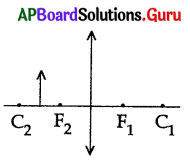A) beyond C2
B) beyond C1
C) at C1
D) at infinityQuestion 88.
A virtual erect image is formed when an object is placed on the principal axis of a convex lens ………………….
A) beyond the centre of curvature
B) at the centre of curvature
C) between centre of curvature and infinity
D) between focal point and optic centre
D) between focal point and optic centre

CONCEPT – III : Lens formula – Lens Mark’s Formula

Question 89.
Which of the following is a lens formula?
A) $$\frac{1}{f}=\frac{1}{v}-\frac{1}{u}$$
B) $$\frac{1}{\mathrm{f}}=\frac{1}{\mathrm{v}}+\frac{1}{\mathrm{u}}$$
C) $$\frac{1}{v}=\frac{1}{f}-\frac{1}{u}$$
D) $$\frac{1}{u}=\frac{1}{v}+\frac{1}{f}$$
A) $$\frac{1}{f}=\frac{1}{v}-\frac{1}{u}$$

Question 90.
Which of the followingis a lens maker’s formula?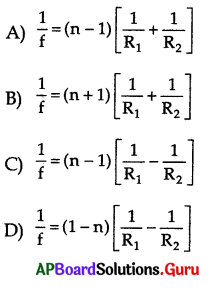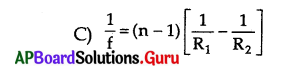Question 91.
The focal length of the lens …………………
A) does not depend upon the surrounding medium
B) depends upon the surrounding medium
C) depends on object distance
D) depends on image distance
B) depends upon the surrounding medium

Question 92.
The focal length of the lens ……………. in water
A) decreases
B) increases
C) remains the same
D) first decreases and then increases
B) increases

Question 93.
Statement X: The Convex lens behaves as a converging lens if it is kept in a medium with refractive index less than the refractive index of the lens.
Statement Y: The convex lens behaves as a diverging lens if it is kept in a medium with refractive index more than the refractive index of the lens.
A) Both statements are true
B) Both statements are false
C) X is true, Y is false
D) X is false, Y is true
B) Both statements are false

Question 94.
An air bubble in water behaves like a ……………………
A) converging lens
B) diverging lens
C) convex lens
D) none
B) diverging lensQuestion 95.
The focal length of double concave lens kept in air with two spherical surfaces of radii R1 = 30 cm and R2 = 60 cm
A) 40 cm
B) – 40 cm
C) 20 cm
D) – 20 cm
B) – 40 cm

Question 96.
Assertion (A): A person standing on the land appears taller than his actual height to a fish inside a pond
Reason (R): Light bends away from the normal as it enters air from water.
A) Both A and R are true and R is the correct explanation of A
B) Both A and R are true and R is not the correct explanation of A
C) A is true but R is false
D) Both A and R are false
E) A is false but R is true
B) Both A and R are true and R is not the correct explanation of A

Question 97.
A double convex lens has two surfaces of equal radii “R” and refractive index n = 1.5. The focal length is …………………..
A) -R
B) +R
C) O
D) $$\frac{1}{2}$$
B) +R

Question 98.
The refractive index of the glass which is symmetrical convergent lens if its focal length is equal to the radius of curvature of its surface ………………..
A) 1
B) $$\frac{2}{3}$$
C) $$\frac{3}{2}$$
D) $$\frac{-3}{2}$$
C) $$\frac{3}{2}$$

Question 99.
The radii of curvature of a double convex lens are 10 cm and 10 cm. Its refractive index is 1.5. The focal length is ……………….
A) 10 cm
B) 0.1 cm
C) 0.01 cm
D) zero
A) 10 cm

Question 100.
The number of images formed by a lens made up of three different material is ………………..
A) 1
B) 2
C) 3
D) 4
C) 3

Question 101.
When a lens of focal length f is cut in to two equal halves perpendicular to principal axis, then the focal length of each part of the lens is ………………
A) remains same
B) double
C) halved
D) None of these
B) doubleQuestion 102.
The focal length of the piano – convex lens is 2R. Its radius of curvature is R then the refractive index of the material used is ………………
A) 2
B) 1.5
C) $$\frac{1}{2}$$
D) 1
B) 1.5

Question 103.
Focal length of the piano – convex lens is ………………… when its radius of curvature is R and n is refractive index of the lens.
A) $$\frac{-R}{n-1}$$
B) $$\frac{\mathrm{n}-1}{\mathrm{R}}$$
C) $$\frac{\mathrm{R}}{\mathrm{n}-1}$$
D) $$\frac{\mathrm{n}-1}{-\mathrm{R}}$$
C) $$\frac{\mathrm{R}}{\mathrm{n}-1}$$

Question 104.
Pick the correct answer from the following two statements.
a) Focal length of a lens depends on the surrounding medium
b) Focal length of a lens changes with the object distance
A) both, (a) and (b) are true
B) both (a) and (b) are false
C) only (a) is true
D) only (b) is true
C) only (a) is true

Question 105.
If 40 cm each is the object and image distance respectively for a convex lens then the focal length is ……………………
A) 80 cm
B) 40 cm
C) 20 cm
D) 25 cm
C) 20 cm

Question 106.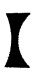Name of the lens shown in the figure is ……………..
A) bi convex lens
B) bi concave lens
C) concavo – convex lens
D) piano – convex lens
B) bi concave lens

Question 107.
If a convex lens has its ob j ect and image distance equal (say x) the focal length is equal to …………………
A) x
B) $$\frac{x}{2}$$
C) $$\frac{2}{x}$$
D) 0
B) $$\frac{x}{2}$$

Question 108.
If the image distance of a convex lens of focal length 25 cm is 75 cm then the object distance is ……………………
A) -37.5 cm
B) 37.5 cm
C) 50 cm
D) -50 cm
A) -37.5 cmQuestion 109.
An image is formed at a distance of 60 cm from the centre of a convex lens when the object distance
is 30 cm. The focal length of the lens is ……………….
A) 90 cm
B) 20 cm
C) 2 cm
D) 0.05 cm
B) 20 cm

Question 110.
An image is formed at distance of 30 cm from the centre of convex lens of focal length 15 cm. The distance of the object is ………………..
A) 2 cm
B) 15 cm
C) 30 cm
D) 45 cm
C) 30 cm

Question 111.
Air bubble in water behaves like a diverging lens because ……………………
A) refractive index of air is less than the refractive index of water
B) air bubble is spherical
C) air bubble is not a perfect sphere
D) None of these
A) refractive index of air is less than the refractive index of water

Question 112.
A magnifying glass forms ……………….
A) a real and diminished image
B) a real and magnified image
C) a virtual and magnified image
D) a virtual and diminished image
C) a virtual and magnified image

Question 113.
Among the following cases the image formed by a convex lens is real.
A) f < u < 2f
B) 2f < u < ∝
C) at 2f
D) all
D) all

Question 114.
Among the following cases the image formed by a convex lens is not real.
A) 0 < u < f
B) f < u < 2f
C) 2f < u < ∝
D) at 2f
A) 0 < u < f

Question 115.
A convex lens of focal length 20 cm is cut into two halves along principal axis then focal length of each part will be …………………
A) 20 cm
B) 10 cm
C) 40 cm
D) 60 cm
A) 20 cm

Question 116.
A convex lens of focal length 20 cm is cut into two halves perpendicular to the principal axis then focal length of each part will be ……………….
A) 20 cm
B) 10 cm
C) 40 cm
D) 60 cm
C) 40 cmQuestion 117.
We can’t discuss the size and nature of the image formed while using a convex lens, when the
object is placed ………………………
A) in between F and C
B) at F
C) between F and optic centre
D) beyond 2F
B) at F

Question 118.
For a given spherical lens the paraxial rays …………………
A) may converge at focus
B) may diverge from focus
C) do not deviate from their path
D) both A and B
D) both A and B

Question 119.
Suppose you are inside the water in a swimming pool near an edge. A friend is standing on the edge. Then your friend will appear
A) taller than his actual height
B) shorter than his actual height
C) actual height
D) both A and B